AP Chemistry : pH

Example Questions

1 2 4 Next →

Example Question #31 : P H

Find the pH of a 0.2M calcium hydroxide solution.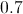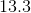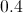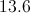Explanation:

Since every calcium hydroxide molecule will dissociate and form two hydroxide ions, the concentration of the hydroxide ions will be twice as much as the initial concentration of the base.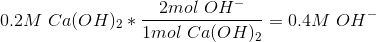This means that the concentration of hydroxide ions in the solution will be 0.4M.We can use this concentration to solve for the pOH fo the solution.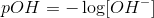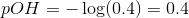Since we are looking for the pH of the solution, we simply subtract the pOH form 14.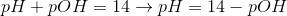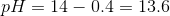This results in a pH of 13.6.

Example Question #32 : P H

Which of the following is a possible pH value for a basic solution?None of theseExplanation:

Basic solutions have a pH level greater than 7, which is the neutral pH. Acidic solutions have a pH level lower than 7.

Example Question #33 : P H

Which of the following is considered a neutral pH?Explanation:

Basic solutions have a pH level greater than 7 and acidic solutions have a pH level lower than 7. A pH of 7 corresponds to a neutral solution.

Example Question #34 : P H

A chemist has an unknown solution. He think the solution is an acid. Which of the following pH's would support his hypothesis?None of theseExplanation:

Acidic solutions have a pH level lower than 7, which is the neutral pH. Basic solutions have a pH level greater than 7.

Example Question #35 : P H

Knowing that the acid ionization constants of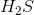are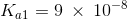and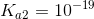, a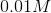solution of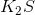will:

have a pH greater than 7

have an acidic pH

exhibit a neutral pH becauseis a salt

have a pH greater than 7

Explanation:

Potassium sulfide is soluble in water yielding the specie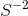which undergoes a two steps basic hydrolysis: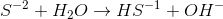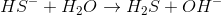The respective hydrolysis constants are: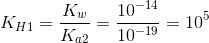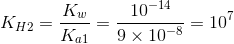Analyzing the rounded values of both hydrolysis constants, we can see that the value of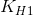is very large then we can assume the first reaction occurs to completion and controls the concentration of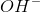in the solution which will be. Hence, the pH will be: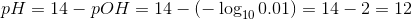Example Question #36 : P H

Consider the following reaction of acetic acid: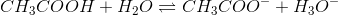The pKa for this reaction is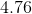. At what pH would the concentrations of the acidic and basic forms of acetic acid be equal to each other?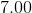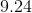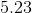Explanation:

This question is presenting us with a reversible chemical reaction of a weak acid. It provides us with the pKa of the acid, and asks us to determine where on the pH spectrum will the acidic form of acetic acid be equal to its basic form.

There is a very important concept that this question highlights, which is that a compound will always have equal amounts of its acidic and basic forms at a pH that is equal to its pKa. This can be shown mathematically by using the Henderson-Hasselbalch equation.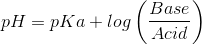As the above equation shows, when the acidic and basic forms are equal, their ratio is equal to. And the logarithm ofis equal to. Thus, what we're left with is: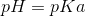1 2 4 Next →